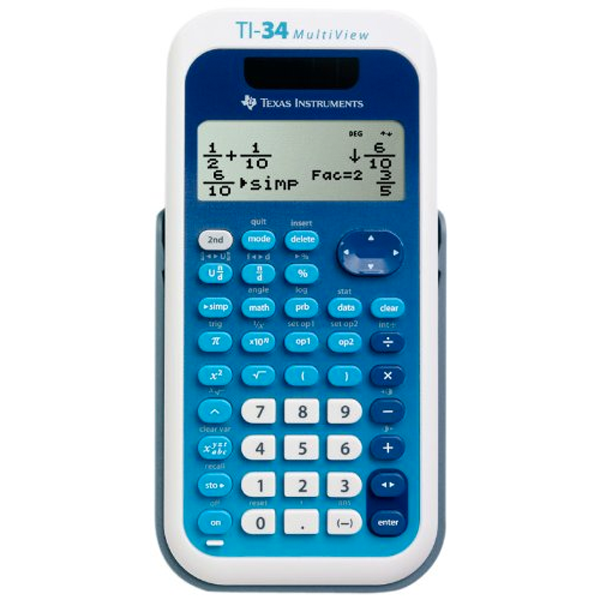# TI-34 MultiView Scientific CalculatorSKU:
1238437

# TI-34 MultiView Scientific Calculator

 \$21.95
Description:

### Key features:

• Four-line display
• One- and two-variable statistics
• MathPrint feature
• Step-by-step fraction simplification
• Edit, cut, and paste entries
• Solar and battery powered
• Exam acceptance: The TI-34 MultiView scientific calculator is approved for use on SAT, ACT, and AP exams.

### Ideal for:

• Middle School math
• Pre-Algebra
• Algebra 1 and 2
• General science
• Geometry

• View more calculations at a time: Four-line display allows you to enter more than one calculation, compare results and explore patterns, all on the same screen.
• MathPrint feature: Use the MathPrint feature to display expressions, symbols, and fractions just as they appear in textbooks.
• Explore fractions: Explore fraction simplification, integer division and constant operators.
• Investigate patterns: Explore patterns via list conversions to see different number formats like decimal, fraction and percent side-by-side.
• SKU:
1238437
Description:

### Key features:

• Four-line display
• One- and two-variable statistics
• MathPrint feature
• Step-by-step fraction simplification
• Edit, cut, and paste entries
• Solar and battery powered
• Exam acceptance: The TI-34 MultiView scientific calculator is approved for use on SAT, ACT, and AP exams.

### Ideal for:

• Middle School math
• Pre-Algebra
• Algebra 1 and 2
• General science
• Geometry